# Solve the inequality 22 +2 - 2 22 - 5.0 + 6 <0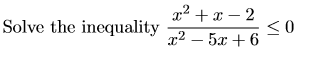Solve the inequality 22 +2 - 2 22 - 5.0 + 6 <0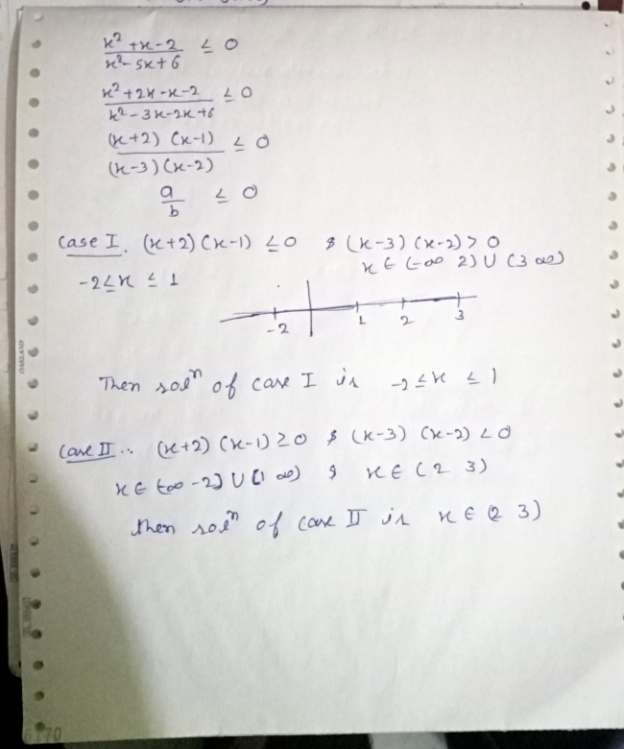##### Add Answer of: Solve the inequality 22 +2 - 2 22 - 5.0 + 6 <0
Similar Homework Help Questions
• ### PLEASE WRITE NEATLY!!! Solve the inequality 22 +2 - 2 22 - 5.0 + 6 <0PLEASE WRITE NEATLY!!! Solve the inequality 22 +2 - 2 22 - 5.0 + 6 <0

• ### Solve the inequality. 2x + 3 -1< 5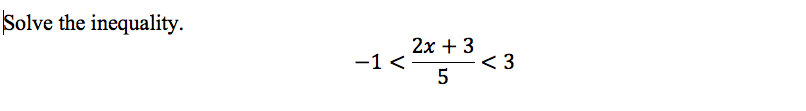Solve the inequality. 2x + 3 -1< 5

• ### Solve y'' +9y = \$(t – 6), y(0) = y'(0) = 0 g(t) = for t...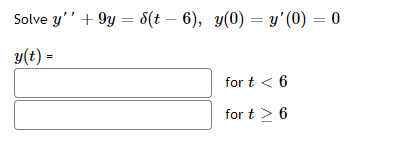Solve y'' +9y = \$(t – 6), y(0) = y'(0) = 0 g(t) = for t < 6 for t > 6

• ### Solve the inequality log2 (x2 – 3x + 4) <1. Give the answer as an interval.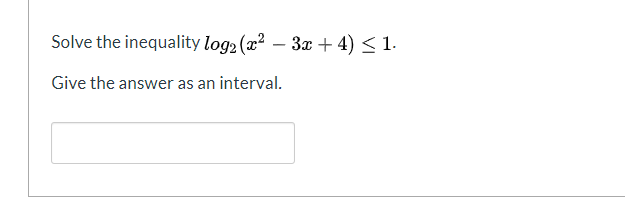Solve the inequality log2 (x2 – 3x + 4) <1. Give the answer as an interval.

• ### Find the Laplace transform of the given function Solve the integral equation f(t) = { 0...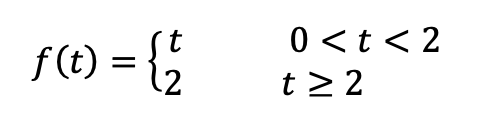Find the Laplace transform of the given function Solve the integral equation f(t) = { 0 < t < 2 t 22 t y(t) = 4t – 3 y(z)sin(t – z)dz 0

• ### Solve the follwing rational inequality: 2 – 3 < 0 x + 1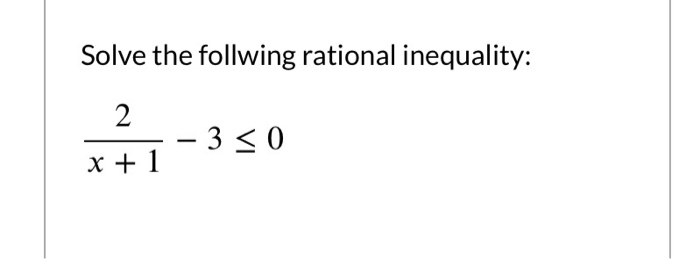Solve the follwing rational inequality: 2 – 3 < 0 x + 1

• ### Solve y'' + 4y = \$(t – 6), y(0) = y'(0) = 0 g(t) = for...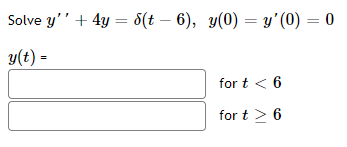Solve y'' + 4y = \$(t – 6), y(0) = y'(0) = 0 g(t) = for t < 6 fort > 6

• ### Solve the inequality f(x) <0, where f(x) = - x2(x + 4), by using the graph...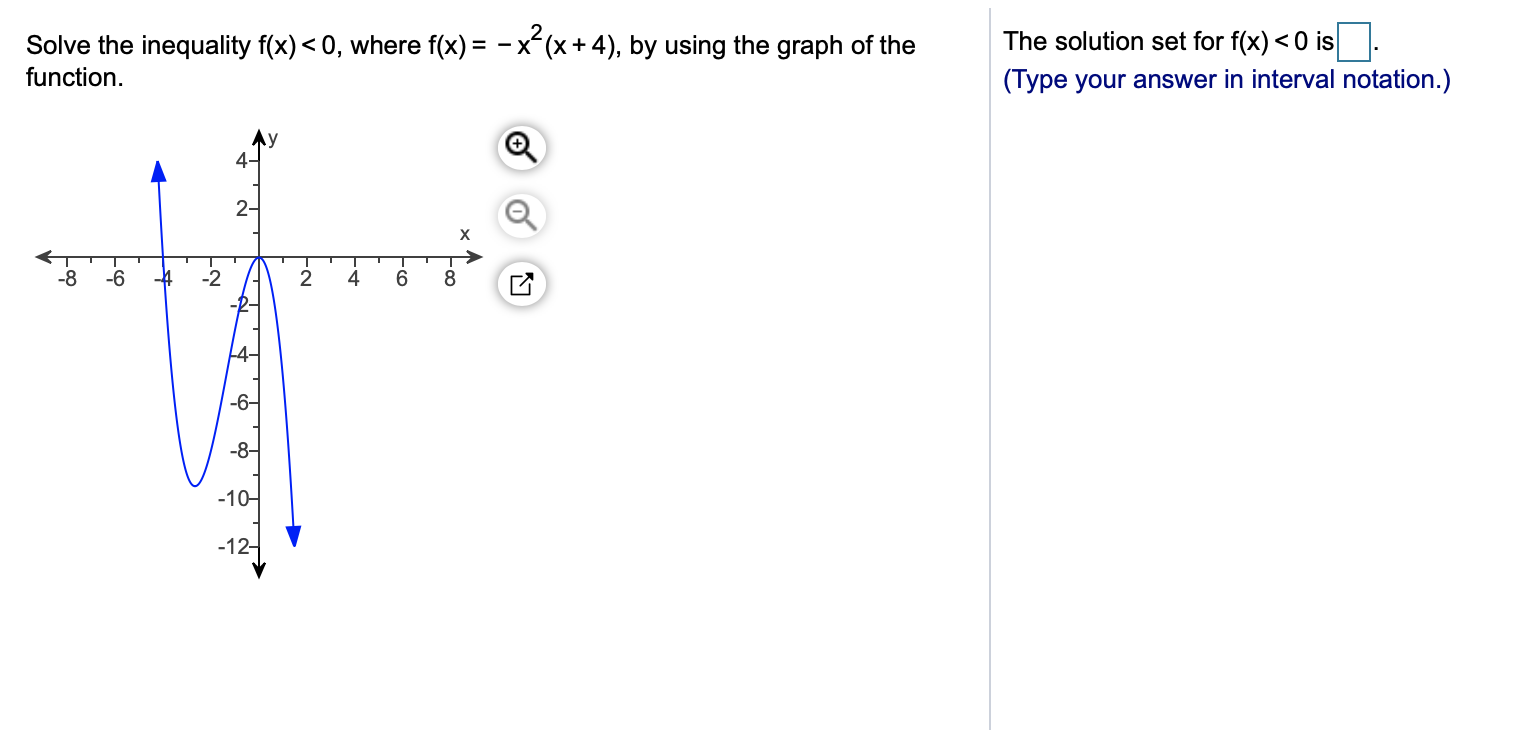Solve the inequality f(x) <0, where f(x) = - x2(x + 4), by using the graph of the The solution set for f(x) <0 is. (Type your answer in interval notation.) function. Ay 4- 2- х 500 -8 -6 -4 -2 2 4. 6 -8- -104 -12-

• ###  1. Solve the first-order equation Tư + (x – 2) = -xe-3, > 0.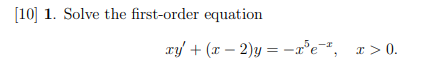1. Solve the first-order equation Tư + (x – 2) = -xe-3, > 0.

• ### Laplace transform of the unit step function y"+y= St/2, if 0 St<6, 13, if t >...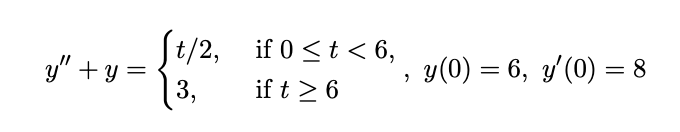Laplace transform of the unit step function y"+y= St/2, if 0 St<6, 13, if t > 6 y(0) = 6, y'(0) = 8

Free Homework App